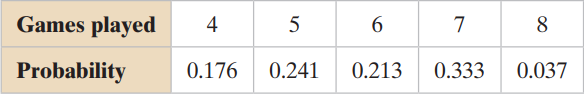×
Get Full Access to Elementary Statistics: Picturing The World - 6 Edition - Chapter 4.1 - Problem 30e
Get Full Access to Elementary Statistics: Picturing The World - 6 Edition - Chapter 4.1 - Problem 30e

×

# Solved: Finding the Mean, Variance, and Standard DeviationISBN: 9780321911216 66

## Solution for problem 30E Chapter 4.1

Elementary Statistics: Picturing the World | 6th Edition

• Textbook Solutions
• 2901 Step-by-step solutions solved by professors and subject experts
• Get 24/7 help from StudySoup virtual teaching assistantsElementary Statistics: Picturing the World | 6th Edition

4 5 1 398 Reviews
31
2
Problem 30E

Finding the Mean, Variance, and Standard Deviation In Exercise, (a) find the mean, variance, and standard deviation of the probability distribution, and (b) interpret the results.

Baseball The number of games played in the World Series from 1903 to 2012Step-by-Step Solution:

Step 1 of 2

Given the number of games played in the World Series from 1903 to 2012.

Then the table is given below.

 Games played Probability 4 0.176 5 0.241 6 0.213 7 0.333 8 0.037

a).  Here we have to find the mean, variance and standard deviation.0.704+1.205+1.278+2.331+0.2965.814

Therefore, the mean is 5.814.

Then the variance is1.391

Then the standard deviation is the square root of the variance.Therefore, the standard deviation is 1.1794.

Step 2 of 2

##### ISBN: 9780321911216

Elementary Statistics: Picturing the World was written by and is associated to the ISBN: 9780321911216. This textbook survival guide was created for the textbook: Elementary Statistics: Picturing the World , edition: 6. The answer to “?Finding the Mean, Variance, and Standard Deviation In Exercise, (a) find the mean, variance, and standard deviation of the probability distribution, and (b) interpret the results.Baseball The number of games played in the World Series from 1903 to 2012” is broken down into a number of easy to follow steps, and 39 words. The full step-by-step solution to problem: 30E from chapter: 4.1 was answered by , our top Statistics solution expert on 08/25/17, 09:43AM. This full solution covers the following key subjects: mean, deviation, variance, standard, Probability. This expansive textbook survival guide covers 66 chapters, and 3049 solutions. Since the solution to 30E from 4.1 chapter was answered, more than 14482 students have viewed the full step-by-step answer.

## Discover and learn what students are asking

Calculus: Early Transcendental Functions : Slope Fields and Eulers Method
?In Exercises 1-8, verify the solution of the differential equation. Solution D

Calculus: Early Transcendental Functions : Conservative Vector Fields and Independence of Path
?In Exercises 1 - 4, show that the value of $$\int_{C} F \cdot d r$$ is the same for each parametric representation of C. \(\mathbf{F}(x, y)=y

Statistics: Informed Decisions Using Data : Inference about Two Population Standard Deviations
?In Problems 9–14, assume that the populations are normally distributed. Test the given hypothesis. 9. s1 ? s2 at the a = 0.05 level of signif

Unlock Textbook Solution

Enter your email below to unlock your verified solution to:

Solved: Finding the Mean, Variance, and Standard Deviation